PHYSICS

Mass

MASS

MA. Fundamental of Mass MB. Kong Frequency and Kong Wavelength MC. Annihilation and Pair Production

MD. Kong Equation

ME. Kong Atom Model MF. Quantum of Atom MG. Perturbation of Photon

MH. Periodic Table

MI. Chemical Reaction MJ. Superconductor MK. Particles and Waves ML. Nuclear Physics

MH. THE PERIODIC TABLE

1. Filling Up the Electrons 2. Periodic Table 3. Group of Vertical Periods

4. Colorful Transition Metals

INTRODUCTION

The Kong Atom Model is well applied to the elements in the periodic table, in describing the chemical properties of elements. According to the properties of the elements across the periodic table, the elements at the same column (vertical periods) possess the same chemical characteristic. Therefore, the vertical periods shall have the same arrangement of reactive electrons.

Base on the addressing method described in the Quantum of Atom, each element in the periodic table is assigned with a set of address of electrons. The addresses are used to explain the chemical properties of the elements.

OBJECTIVES

1)      To assign with a set of electrons address to each element in the periodic table.

2)      To tabulate each element in the periodic table in the format of electron addresses.

3)      To describe the property of the vertical periods.

4)      To describe the colorful transition metal based on the state energy.

MH.1.0        FILLING UP THE ELECTRONS

In filling up the electrons to a bare atom, the orbital are always filled in the order of increasing energy. As described in the chapter “Quantum of Atom”, the populations of the orbital depend on the orbital quantum numbers, which are the principle orbital number (PON), n, and the sub-orbital number (SON), l. Each sub-orbital maximum can be filled with 2 numbers of electrons, Ne. An example of the electron filling sequence is listed below:

 Orbit, n Sub-orbit, l £ n Numbers of sub-orbital, ln = (2n – 1) Population, Ne = 2ln 1 1 1 2 2 2, 1 3 6 3 3, 2, 1 5 10 4 4, 3, 2, 1 7 14 5 5, 4, 3, 2, 1 9 18

Table MH.1.1

This filling sequence will be used to address the electrons to each element in periodic table.

In this addressing method, for elements with high proton number, the electrons may stay in the higher PON with lower SON instead of higher SON of current PON due to the energy level. The electrons may not fully fill up the lower PON.

MH.2.0        PERIODIC TABLE

In periodic table, the elements at each column possess the same characteristic. This is due to the reactive group of the electron valence. Therefore, each element at each column shall have the same profile of the electron allocation. The periodic table is shown in table MH.2.1. Table MH.2.1 suggests the electron addresses and allocations for each element. The bold value shown in table MH.2.1 is the suggested group of reactive electrons. The reactive group of electrons determines the chemical properties of elements. However, the actual addresses of the substances in the periodic table are still subjected to change, pending for more information.

MH.2.1        The Basic Group of Elements in Periodic Table

 PERIODIC TABLE FOR 8 BASIC GROUP OF ELEMENTS Legend GROUP I Z Proton Number GROUP VIII 1 E Element 2 H n = Principle Orbital Number He n = 1 l = Sub-Orbitals Number n = 1 l = (1) Ne = Numbers of Electrons l = (1) Ne = (1) Ne = 2 GROUP II GROUP III GROUP IV GROUP V GROUP VI GROUP VII 3 4 5 6 7 8 9 10 Li Be B C N O F Ne n = 2, 1 n = 2, 1 n = 2, 1 n = 2, 1 n = 2, 1 n = 2, 1 n = 3, 2, 1 n = 3, 2, 1 l = (1), (1) l = (1), (1) l = (3), (1) l = (3), (1) l = (3), (1) l = (3), (1) l = (1), (3), (1) l = (1), (3), (1) Ne = (1)+(2) Ne = (2)+(2) Ne = (3)+(2) Ne = (4)+(2) Ne = (5)+(2) Ne = (6)+(2) Ne = (1)+(6)+(2) Ne = (2)+(6)+(2) 11 12 13 14 15 16 17 18 Na Mg Al Si P S Cl Ar n = 3, 2, 1 n = 3, 2, 1 n = 3, 2, 1 n = 3, 2, 1 n = 3, 2, 1 n = 3, 2, 1 n = 4, 3, 2, 1 n = 4, 3, 2, 1 l = (2), (3), (1) l = (2), (3), (1) l = (3), (3), (1) l = (3), (3), (1) l = (4), (3), (1) l = (4), (3), (1) l = (1), (4), (3), (1) l = (1), (4), (3), (1) Ne = (3)+(6)+(2) Ne = (4)+(6)+(2) Ne = (5)+(6)+(2) Ne = (6)+(6)+(2) Ne = (7)+(6)+(2) Ne = (8)+(6)+(2) Ne = (1)+(8)+(6)+(2) Ne = (2)+(8)+(6)+(2) 19 20 31 32 33 34 35 36 K Ca Ga Ge As Se Br Kr n = 4, 3, 2, 1 n = 4, 3, 2, 1 n = 5, 4, 3, 2, 1 n = 5, 4, 3, 2, 1 n = 5, 4, 3, 2, 1 n = 5, 4, 3, 2, 1 n = 6, 5, 4, 3, 2, 1 n = 6, 5, 4, 3, 2, 1 l = (2), (4), (3), (1) l = (2), (4), (3), (1) l = (3), (5), (4), (3), (1) l = (3), (5), (4), (3), (1) l = (4), (5), (4), (3), (1) l = (4), (5), (4), (3), (1) l = (1), (4), (5), (4), (3), (1) l = (1), (4), (5), (4), (3), (1) Ne = (3)+(8)+(6)+(2) Ne = (4)+(8)+(6)+(2) Ne = (5)+(10)+(8)+(6)+(2) Ne = (6)+(10)+(8)+(6)+(2) Ne = (7)+(10)+(8)+(6)+(2) Ne = (8)+(10)+(8)+(6)+(2) Ne = (1)+(8)+(10)+(8)+(6)+(2) Ne = (2)+(8)+(10)+(8)+(6)+(2) 37 38 49 50 51 52 53 54 Rb Sr In Sn Sb Te I Xe n = 6, 5, 4, 3, 2, 1 n = 6, 5, 4, 3, 2, 1 n = 6, 5, 4, 3, 2, 1 n = 6, 5, 4, 3, 2, 1 n = 6, 5, 4, 3, 2, 1 n = 6, 5, 4, 3, 2, 1 n = 7, 6, 5, 4, 3, 2, 1 n = 7, 6, 5, 4, 3, 2, 1 l = (2), (4), (5), (4), (3), (1) l = (2), (4), (5), (4), (3), (1) l = (3), (6), (7), (5), (3), (1) l = (3), (6), (7), (5), (3), (1) l = (4), (6), (7), (5), (3), (1) l = (4), (6), (7), (5), (3), (1) l = (1), (4), (6), (7), (5), (3), (1) l = (1), (4), (6), (7), (5), (3), (1) Ne = (3)+(8)+(10)+(8)+(6)+(2) Ne = (4)+(8)+(10)+(8)+(6)+(2) Ne = (5)+(12)+(14)+(10)+(6)+(2) Ne = (6)+(12)+(14)+(10)+(6)+(2) Ne = (7)+(12)+(14)+(10)+(6)+(2) Ne = (8)+(12)+(14)+(10)+(6)+(2) Ne = (1)+(8)+(12)+(14)+(10)+(6)+(2) Ne = (2)+(8)+(12)+(14)+(10)+(6)+(2) 55 56 81 82 83 84 85 86 Cs Ba Tl Pb Bi Po At Rn n = 7, 6, 5, 4, 3, 2, 1 n = 7, 6, 5, 4, 3, 2, 1 n = 8, 7, 6, 5, 4, 3, 2, 1 n = 8, 7, 6, 5, 4, 3, 2, 1 n = 8, 7, 6, 5, 4, 3, 2, 1 n = 8, 7, 6, 5, 4, 3, 2, 1 n = 9, 8, 7, 6, 5, 4, 3, 2, 1 n = 9, 8, 7, 6, 5, 4, 3, 2, 1 l = (2), (4), (6), (7), (5), (3), (1) l = (2), (4), (6), (7), (5), (3), (1) l = (3), (6), (7), (9), (7), (5), (3), (1) l = (3), (6), (7), (9), (7), (5), (3), (1) l = (4), (6), (7), (9), (7), (5), (3), (1) l = (4), (6), (7), (9), (7), (5), (3), (1) l = (1), (4), (6), (7), (9), (7), (5), (3), (1) l = (1), (4), (6), (7), (9), (7), (5), (3), (1) Ne = (3), (8)+(12)+(14)+(10)+(6)+(2) Ne = (4)+(8)+(12)+(14)+(10)+(6)+(2) Ne = (5)+(12)+(14)+(18)+(14)+(10)+(6)+(2) Ne = (6)+(12)+(14)+(18)+(14)+(10)+(6)+(2) Ne = (7)+(12)+(14)+(18)+(14)+(10)+(6)+(2) Ne = (8)+(12)+(14)+(18)+(14)+(10)+(6)+(2) Ne = (1)+(8)+(12)+(14)+(18)+(14)+(10)+(6)+(2) Ne = (2)+(8)+(12)+(14)+(18)+(14)+(10)+(6)+(2) Table MH.2.1A

MH.2.2        The Transition Metals Group

 PERIODIC TABLE FOR TRANSITION METALS 21 22 23 24 25 26 27 28 29 30 Sc Ti V Cr Mn Fe Co Ni Cu Zn n = 4, 3, 2, 1 n = 4, 3, 2, 1 n = 4, 3, 2, 1 n = 4, 3, 2, 1 n = 4, 3, 2, 1 n = 5, 4, 3, 2, 1 n = 5, 4, 3, 2, 1 n = 5, 4, 3, 2, 1 n = 5, 4, 3, 2, 1 n = 5, 4, 3, 2, 1 l = (3), (4), (3), (1) l = (3), (4), (3), (1) l = (4), (4), (3), (1) l = (4), (4), (3), (1) l = (5), (4), (3), (1) l = (1), (4), (4), (3), (1) l = (2), (4), (4), (3), (1) l = (2), (4), (4), (3), (1) l = (2), (5), (4), (3), (1) l = (2), (5), (4), (3), (1) Ne = (5)+(8)+(6)+(2) Ne = (6)+(8)+(6)+(2) Ne = (7)+(8)+(6)+(2) Ne = (8)+(8)+(6)+(2) Ne = (9)+(8)+(6)+(2) Ne = (2)+(8)+(8)+(6)+(2) Ne = (3)+(8)+(8)+(6)+(2) Ne = (4)+(8)+(8)+(6)+(2) Ne = (3)+(10)+(8)+(6)+(2) Ne = (4)+(10)+(8)+(6)+(2) 39 40 41 42 43 44 45 46 47 48 Y Zr Nb Mo Tc Ru Rh Pd Ag Cd n = 6, 5, 4, 3, 2, 1 n = 6, 5, 4, 3, 2, 1 n = 6, 5, 4, 3, 2, 1 n = 6, 5, 4, 3, 2, 1 n = 6, 5, 4, 3, 2, 1 n = 6, 5, 4, 3, 2, 1 n = 6, 5, 4, 3, 2, 1 n = 6, 5, 4, 3, 2, 1 n = 6, 5, 4, 3, 2, 1 n = 6, 5, 4, 3, 2, 1 l = (1), (3), (7), (5), (3), (1) l = (1), (3), (7), (5), (3), (1) l = (1), (4), (7), (5), (3), (1) l = (1), (4), (7), (5), (3), (1) l = (1), (5), (7), (5), (3), (1) l = (1), (5), (7), (5), (3), (1) l = (2), (5), (7), (5), (3), (1) l = (2), (5), (7), (5), (3), (1) l = (2), (6), (7), (5), (3), (1) l = (2), (6), (7), (5), (3), (1) Ne = (2)+(5)+(14)+(10)+(6)+(2) Ne = (2)+(6)+(14)+(10)+(6)+(2) Ne = (2)+(7)+(14)+(10)+(6)+(2) Ne = (2)+(8)+(14)+(10)+(6)+(2) Ne = (2)+(9)+(14)+(10)+(6)+(2) Ne = (2)+(10)+(14)+(10)+(6)+(2) Ne = (3)+(10)+(14)+(10)+(6)+(2) Ne = (4)+(10)+(14)+(10)+(6)+(2) Ne = (3)+(12)+(14)+(10)+(6)+(2) Ne = (4)+(12)+(14)+(10)+(6)+(2) 71 72 73 74 75 76 77 78 79 80 Lu Hf Ta W Re Os Ir Pt Au Hg n = 8, 7, 6, 5, 4, 3, 2, 1 n = 8, 7, 6, 5, 4, 3, 2, 1 n = 8, 7, 6, 5, 4, 3, 2, 1 n = 8, 7, 6, 5, 4, 3, 2, 1 n = 8, 7, 6, 5, 4, 3, 2, 1 n = 8, 7, 6, 5, 4, 3, 2, 1 n = 8, 7, 6, 5, 4, 3, 2, 1 n = 8, 7, 6, 5, 4, 3, 2, 1 n = 8, 7, 6, 5, 4, 3, 2, 1 n = 8, 7, 6, 5, 4, 3, 2, 1 l = (1), (3), (7), (9), (7), (5), (3), (1) l = (1), (3), (7), (9), (7), (5), (3), (1) l = (1), (4), (7), (9), (7), (5), (3), (1) l = (1), (4), (7), (9), (7), (5), (3), (1) l = (1), (5), (7), (9), (7), (5), (3), (1) l = (1), (5), (7), (9), (7), (5), (3), (1) l = (2), (5), (7), (9), (7), (5), (3), (1) l = (2), (5), (7), (9), (7), (5), (3), (1) l = (2), (6), (7), (9), (7), (5), (3), (1) l = (2), (6), (7), (9), (7), (5), (3), (1) Ne = (2)+(5)+(14)+(18)+(14)+(10)+(6)+(2) Ne = (2)+(6)+(14)+(18)+(14)+(10)+(6)+(2) Ne = (2)+(7)+(14)+(18)+(14)+(10)+(6)+(2) Ne = (2)+(8)+(14)+(18)+(14)+(10)+(6)+(2) Ne = (2)+(9)+(14)+(18)+(14)+(10)+(6)+(2) Ne = (2)+(10)+(14)+(18)+(14)+(10)+(6)+(2) Ne = (3)+(10)+(14)+(18)+(14)+(10)+(6)+(2) Ne = (4)+(10)+(14)+(18)+(14)+(10)+(6)+(2) Ne = (3)+(12)+(14)+(18)+(14)+(10)+(6)+(2) Ne = (4)+(12)+(14)+(18)+(14)+(10)+(6)+(2) Table MH.2.1B

MH.2.3        The Inner Transition Metals Group

 PERIODIC TABLE FOR INNER TRANSITION METALS GROUP 57 58 59 60 61 62 63 La Ce Pr Nd Pm Sm Eu n = 7, 6, 5, 4, 3, 2, 1 n = 7, 6, 5, 4, 3, 2, 1 n = 7, 6, 5, 4, 3, 2, 1 n = 7, 6, 5, 4, 3, 2, 1 n = 7, 6, 5, 4, 3, 2, 1 n = 7, 6, 5, 4, 3, 2, 1 n = 7, 6, 5, 4, 3, 2, 1 l = (1), (3), (9), (7), (5), (3), (1) l = (1), (3), (9), (7), (5), (3), (1) l = (1), (4), (9), (7), (5), (3), (1) l = (1), (4), (9), (7), (5), (3), (1) l = (1), (5), (9), (7), (5), (3), (1) l = (1), (5), (9), (7), (5), (3), (1) l = (1), (6), (9), (7), (5), (3), (1) Ne = (2)+(5)+(18)+(14)+(10)+(6)+(2) Ne = (2)+(6)+(18)+(14)+(10)+(6)+(2) Ne = (2)+(7)+(18)+(14)+(10)+(6)+(2) Ne = (2)+(8)+(18)+(14)+(10)+(6)+(2) Ne = (2)+(9)+(18)+(14)+(10)+(6)+(2) Ne = (2)+(10)+(18)+(14)+(10)+(6)+(2) Ne = (2)+(11)+(18)+(14)+(10)+(6)+(2) 64 65 66 67 68 69 70 Gd Tb Dy Ho Er Tm Yb n = 7, 6, 5, 4, 3, 2, 1 n = 7, 6, 5, 4, 3, 2, 1 n = 7, 6, 5, 4, 3, 2, 1 n = 8, 7, 6, 5, 4, 3, 2, 1 n = 8, 7, 6, 5, 4, 3, 2, 1 n = 8, 7, 6, 5, 4, 3, 2, 1 n = 8, 7, 6, 5, 4, 3, 2, 1 l = (1), (6), (9), (7), (5), (3), (1) l = (1), (7), (9), (7), (5), (3), (1) l = (1), (7), (9), (7), (5), (3), (1) l = (1), (1), (7), (9), (7), (5), (3), (1) l = (1), (1), (7), (9), (7), (5), (3), (1) l = (1), (2), (7), (9), (7), (5), (3), (1) l = (1), (2), (7), (9), (7), (5), (3), (1) Ne = (2)+(12)+(18)+(14)+(10)+(6)+(2) Ne = (2)+(13)+(18)+(14)+(10)+(6)+(2) Ne = (2)+(14)+(18)+(14)+(10)+(6)+(2) Ne = (2)+(1)+(14)+(18)+(14)+(10)+(6)+(2) Ne = (2)+(2)+(14)+(18)+(14)+(10)+(6)+(2) Ne = (2)+(3)+(14)+(18)+(14)+(10)+(6)+(2) Ne = (2)+(4)+(14)+(18)+(14)+(10)+(6)+(2) Table MH.2.1C

MH.3.0        GROUPS OF VERTICAL PERIODS

As shown in table MH.2.1, in each group of vertical periods, each vertical period has the same number of reactive electrons as highlighted in bold. This suggests the chemical properties of each group. Below describes some of the interesting chemical reaction of the difference groups of the vertical periods.

MH.3.1        Lithium – Group I

Lithium has one electron valence at n = 2. Lithium is reactive to donate the single electron valence to achieve better stability of element Helium.

MH.3.2        Beryllium – Group II

Similarly to element Lithium, Beryllium has two electron valence at n = 2, which are ready to react and donate to achieve better stability of element Helium.

MH.3.3        Boron – Group III

Boron has 3 electron valences that ready for reaction. Due to the tiny size of Boron, it is only mild electropositive. Boron tends to share the electron instead of donate. This causes the element Boron possesses the characteristic of metalloids.

MH.3.4        Carbon – Group IV

Carbon has four electron valences. Carbon is able to perform 4 bonding to achieve the stability of element Neon. A methane molecule structure is suggested in chapter “The Chemical Reaction”, the quantum stage of each chemical bond is illustrated. The pi-bonding of carbon for molecule ethene is also described. The pi-bonding of carbon to carbon atom happen at stage l = 1 for n = 2 and 3. Due to this reason, the double bonding of ethene is aligned.

MH.3.5        Nitrogen – Group V

Nitrogen has 5 electron valences, but only 3 electron valences are ready for reaction to achieve the stability of the element Neon.

MH.3.6        Oxygen – Group VI

Oxygen has the fully filled order at n = 2. However, oxygen atom is not magnetically neutral due to the tilted electrons as described in the “Quantum of Atom”. Therefore, it is reactive by sharing two electron valences in achieving the state of element Neon. Oxygen atom is temporarily stable especially at low temperature. This is the special characteristic of oxygen atom that allow the present of oxygen atom radical in the ozone molecule.

MH.3.7        Fluorine – Group VII

Fluorine in Group VII has only one electron valence at l = 1. Therefore, group VII is electronegative to take another electron to achieve the stability of element Neon.

MH.3.8        Inert Gases – Group VIII

It is shown in table MH.2.1A that the electron valence for each element along this column is the same. It is described that for the group of inert gases, the elements are very stable and inert due to the two electrons at l = 1. Each element possesses two electron valences similar to the element Helium.

As described in the chapter “Quantum of Atom”, electrons located at l = 1 has good stability because of no repulsive force between electrons or the repulsive forces between electrons are balanced. The two electrons valences of inert element travel perpendicular to the direction of angular momentum and magnetic moment of atom, ma. These two perpendicular moving electron valences generate magnetic moment, me at opposite direction. The generated magnetic moment by the electrons is able to 'neutralize' the magnetic moment of the atom and increases the magnetic stability of atom. This phenomenon makes the inert gas element stable.

MH.3.9        Transition Metals

Transition metals are able to produce few different stable ionic stages. This is due to the non-fully filled SON at lower PON. Electrons are able to transit from one SON at lower PON to another SON at higher PON or vice versa. The transitions of electrons produce or absorb photons.

At elevated temperature, electrons may not be able to stay at lowest orbital all the time. Electrons at lower energy level gain energy from the external and jump to higher energy level.

MH.3.9.1        Iron

Iron is a very special element in the periodic table. Iron atom has 26 numbers of electrons surrounding it, with 2 electron valences at n = 5, l = 1. Iron atom is able to form the ionic stage of 2+ by giving out the two electron valences at n = 4, l = 4, but not n = 5, l = 1. However, for ionic stage of iron at 3+, the atom iron losses another one electron valence at n = 5, l = 1. The losses of electron at SON = 1 causes the significant magnetic characteristic of iron molecule. Below suggests the electron address for the following ionic stage of iron.

 Fe2+,     n = 5, 4, 3, 2, 1   l = (1), (3), (4), (3), (1)  Ne = (2)+(6)+(8)+(6)+(2) Fe3+,     n = 5, 4, 3, 2, 1   l = (1), (3), (4), (3), (1)  Ne = (1)+(6)+(8)+(6)+(2)

MH.3.9.2        Chromium

Chromium has 24 numbers of electrons surrounding the atom. Chromium is able to form many different ionic stages such as 6+, 3+ and etcetera. Below suggests the electron address for the following ionic stage.

 Cr6+,     n = 4, 3, 2, 1   l = (1), (4), (3), (1)  Ne = (2)+(8)+(6)+(2)

MH.3.9.3        Manganese

Manganese has 25 numbers of electrons surrounding it. Manganese is able to form many different ionic stages such as 7+, 5+ and etcetera. Below suggests the electron address for the following ionic stage.

 Mn7+,     n = 4, 3, 2, 1   l = (1), (4), (3), (1)  Ne = (2)+(8)+(6)+(2) Mn5+,     n = 4, 3, 2, 1   l = (1), (5), (3), (1)  Ne = (2)+(10)+(6)+(2)

MH.3.9.4       Cobalt

Cobalt has 27 numbers of electrons surrounding it. Cobalt is able to form few different ionic stages such as 2+ and etcetera. Below suggests the electron address for the following ionic stage.

 Co2+,     n = 5, 4, 3, 2, 1   l = (1), (4), (4), (3), (1)  Ne = (2)+(7)+(8)+(6)+(2)

MH.3.9.5       Nickel

Nickel has 28 numbers of electrons surrounding it. Nickel is able to form two different ionic stages such as 2+ and 4+. Below suggests the electron address for the following ionic stage.

 Ni2+,     n = 5, 4, 3, 2, 1   l = (1), (4), (4), (3), (1)  Ne = (2)+(8)+(8)+(6)+(2) Ni4+,     n = 5, 4, 3, 2, 1   l = (1), (3), (4), (3), (1)  Ne = (2)+(6)+(8)+(6)+(2)

MH.3.9.6        Copper

Copper has 29 numbers of electrons surrounding it. Copper is able to form two ionic stages which is 1+ and 2+. Below suggests the electron address for the following ionic stage.

 Cu1+,     n = 5, 4, 3, 2, 1   l = (1), (5), (4), (3), (1)  Ne = (2)+(10)+(8)+(6)+(2) Cu2+,     n = 5, 4, 3, 2, 1   l = (1), (5), (4), (3), (1)  Ne = (2)+(9)+(8)+(6)+(2)

MH.3.9.7       Zinc

Zinc has 30 numbers of electrons surrounding it. Zinc is able to form one ionic stage which is 2+. Below suggests the electron address for the following ionic stage.

 Zn2+,     n = 5, 4, 3, 2, 1   l = (1), (5), (4), (3), (1)  Ne = (2)+(10)+(8)+(6)+(2)

MH.4.0        COLORFUL TRANSITION METALS

From the periodic table, the transition metals have non-fully filled SON at l = 3, 4 and 5. Electrons are able to transit in between these three sub-orbitals.

From the chapter “Kong Atom Model”, the state energy is quote as follow,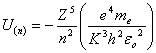… eq. MH.4.1

where   Z = proton number

n = principle orbital number

e = charge of electron

me = mass of electron

K = Kong function

h = Plank's constant

eo = permittivity constant

Again, for electron whose possesses low ionization energy, the electron circulates at low velocity, the Kong function is given as follow,

K = 2Z                                                                                                … eq. MH.4.2

Substitute eq. MH.4.2 into MH.4.1, we obtain the energy of the electron at each quantum state,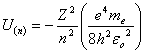… eq. MH.4.3

MH.4.1        Ionic Stage of 2+

For ionic substance with the ionic stage of 2+, the charge of atom is 2. Therefore, substitute Z = 2 into eq. MH.4.3, we obtain,… eq. MH.4.4

Electrons absorb energy from external and jump from PON, n = 3 to 4. Then, for the electron drops from PON, n = 4 to 3, it releases photon energy as follow,

U 2+(4-3) = hf 2+(4-3)                                                                         … eq. MH.4.5

Substitute eq. MH.4.5 into eq. MH.4.4 and substitute n = 4 and 3, we obtain,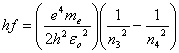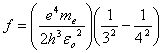… eq. MH.4.6

Substitute in all the constants, the release photon has the frequency of,

f 2+(4-3) = 6.39 x 1014 Hz

and the correspondent wavelength is

l 2+(4-3) = 469 nm                                                                              … eq. MH.4.7

For electrons absorb energy from external and jump from PON, n = 4 to 5. Then, for electron drops from PON, n = 5 to 4, it releases photon energy as follow,

U 2+(5-4) = hf 2+(5-4)                                                                         … eq. MH.4.8

Substitute eq. MH.4.8 into eq. MH.4.4 and substitute n = 5 and 4, we obtain,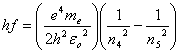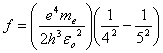… eq. MH.4.9

Substitute in all the constants, the release photon has the frequency of,

f 2+(5-4) = 2.96 x 1014 Hz

and the correspondent wavelength is

l 2+(5-4) = 1013 nm                                                                            … eq. MH.4.10

MH.4.2        Ionic Stage of 3+

For ionic substance with the ionic stage of 3+, the charge of atom is 3. Therefore, substitute Z = 3 into eq. MH.4.3, we obtain,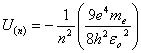… eq. MH.4.11

Electrons absorb energy from external and jump from PON, n = 3 to 4. Then, for electron drops from PON, n = 4 to 3, it releases photon energy as follow,

U 3+(4-3) = hf 3+(4-3)                                                                         … eq. MH.4.12

Substitute eq. MH.4.12 into eq. MH.4.11 and substitute n = 5 and 4, we obtain,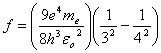… eq. MH.4.13

Substitute in all the constants, the release photon has the frequency of,

f 3+(4-3) = 1.44 x 1015 Hz

and the correspondent wavelength is

l 3+(4-3) = 208 nm                                                                              … eq. MH.4.14

For electrons absorb energy from external and jump from PON, n = 4 to 5. Then, for electron drops from PON, n = 5 to 4, it releases photon energy as follow,

U 3+(5-4) = hf 3+(5-4)                                                                         … eq. MH.4.15

Substitute eq. MH.4.15 into eq. MH.4.11 and substitute n = 5 and 4, we obtain,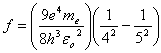… eq. MH.4.16

Substitute in all the constants, the release photon has the frequency of,

f 3+(5-4) = 6.66 x 1014 Hz

and the correspondent wavelength is

l 3+(5-4) = 450 nm                                                                              … eq. MH.4.17

MH.4.3        Colorful Ionic Substances

From the above, for the ionic stage transition metal, the electrons are able to travels in between the non-fully filled PON. The wavelength of the released photon falls in the range between the wavelength of infrared (eq. MH.4.10) and ultraviolet (eq. MH.4.14). The wavelength in between infrared and ultraviolet is the wavelength in the range of visible light.

Ionic transition metals absorb energy from visible light such as sunlight at certain frequency depending on the state energy level of PON. The excited electrons release light at the same frequency. Therefore, ionic substances of the group of transition metals are colorful.

For water hydrated ionic substances, the water molecule has electric dipole and able to reduce the charge of ionic atom. The value of Z in eq. MH.4.3 is reduced for hydrated substances. Different degree of hydration reduces the value of Z differently. Therefore, different degree of hydration on the same ionic substance may produce different type of color.

The released photon wavelength is reverse proportion to the ionic charge to the power of two, which is expressed as follow,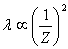… eq. MH.4.18

To compare for two different ionic stages, eq. MH.4.18 can be written as follow,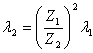… eq. MH.4.19

For example of the ionic substance Fe3+, the wavelength is given by eq. MH.4.17. Due to the tilting effect with some reduction of energy level, the equivalent charge is said roughly 2.65+. Substitute all these information into eq. MH.4.19; we obtain the released photon wavelength for ion Fe3+,… eq. MH.4.20

The ion Fe3+ possesses the color of yellowish orange. Another example on Fe2+, the wavelength is given by eq. MH.4.7. Due to the tilting effect with some reduction of energy level, the equivalent charge is said roughly 1.85+. Substitute all these information into eq. MH.4.19; we obtain the wavelength of ion Fe2+,… eq. MH.4.21

The ion Fe2+ possesses the color of yellowish green.

DISCUSSIONS AND CONCLUSIONS

The elements in periodic table are given the electron addresses. Each vertical period have the same electrons configuration. This tells that the property of elements at the same column has similarity.

Elements within the group of transition metal are able to produce few ionic stages. This is due to the non-fully filled sub-orbital. The ionic stages for substances of transition metals are colorful due to the transition of electrons from high to low energy level. The transitions of electrons release photon energy at the range of visible wavelength.

For ionic transition metals, the sub-orbital or the tilting effect of electron orbits slightly reduces the principle orbital energy level. The photon wavelength or the color of ion shifts from violet towards red color.

This website is originated on 15-Mar-2007,

updated on 4-Jan-2009.